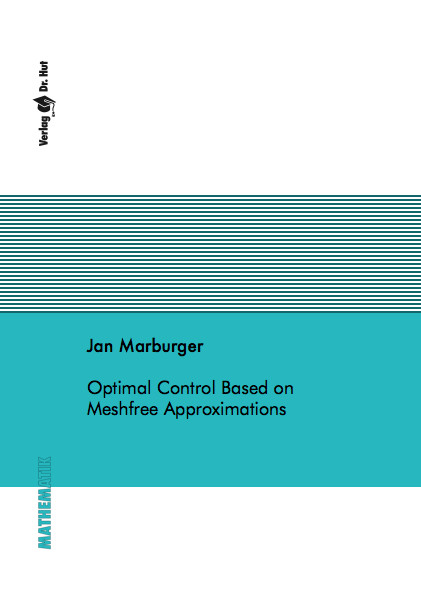Datenbestand vom 20. August 2019aktualisiert am 20. August 2019

# ISBN 9783843900782Euro 60,00 inkl. 7% MwSt

978-3-8439-0078-2, Reihe Mathematik

Jan Marburger
Optimal Control Based on Meshfree Approximations

147 Seiten, Dissertation Technische Universität Kaiserslautern (2011), Softcover, A5

## Zusammenfassung / Abstract

In this thesis we investigate optimal control problems based on meshfree or particle methods. After a short introduction to the functional analysis needed to investigate optimal control problems and the theory and application of particle methods, we consider the optimisation of 1D hyperbolic equations using a particle method based on a delta approximation. Here, we compare the main optimisation approaches, that is, "first optimise, then discretise" and "first discretise, then optimise". Additionally, a "first transform, then optimise, then discretise" approach is considered. We show the equivalence, up to the discretisation error, of all three approaches and verify it numerically. Then an error estimate for the optimal control of the one dimensional viscous Burgers equation based on a delta approximation is derived. First the continuous control problem is derived and investigated. Second the convergence of the discretised state and adjoint equation to the corresponding continuous equations is considered. We also show the convergence of the optimal control function obtained by the discrete optimisation to the continuous one. These results are confirmed numerically. Finally, we investigate numerical approaches for varying domain problems in two dimensions. First, we consider a moving domain application, where the heat distribution in a melt drop, whose shape is already known, is optimised. In contrast to this case, the domain of free surface problems depends on the solution itself, for instance a water surface depends on the flow velocity. After a short introduction to this kind of problem and the difficulties which occur in optimisation, we derive a simplified approach, which takes advantage of the structure of particle methods. This strategy is verified numerically for the optimisation of a liquid tank, involving the Navier-Stokes equation, and the melting/solidification of a material which yields a Stefan-type problem.